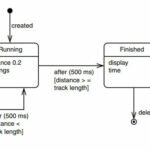# Static Functions in C++ – theteche.com

In the STATIC example in before chapter, “Objects and Classes,” we introduced static data members. As you may recall, a static data member is not duplicated for each object; rather a single data item is shared by all objects of a class. The STATIC example showed a class that kept track of how many objects of itself there were. Let’s extend this concept by showing how functions as well as data may be static.

Besides showing static functions, our example will model a class that provides an ID number for each of its objects. This allows you to query an object to find out which object it is a capability that is sometimes useful in debugging a program, among other situations. The program also casts some light on the operation of destructors. Here’s the listing for STATFUNC:

```// statfunc.cpp
// static functions and ID numbers for objects
#include  <iostream>
using namespace std;
////////////////////////////////////////////////////////////////
class gamma
{
private:
static int total; //total objects of this class
// (declaration only)
int id; //ID number of this object
public:
gamma() //no-argument constructor
{
id = total; //id equals current total
}
~gamma() //destructor
{
total--;
cout << “Destroying ID number “ << id << endl;
}
static void showtotal() //static function
{
cout << “Total is “ << total << endl;
}
void showid() //non-static function
{
cout << “ID number is “ << id << endl;
}
};
//--------------------------------------------------------------
int gamma::total = 0; //definition of total
////////////////////////////////////////////////////////////////
int main()
{
gamma g1;
gamma::showtotal();
gamma g2, g3;
gamma::showtotal();
g1.showid();
g2.showid();
g3.showid();
cout << “----------end of program----------\n”;
return 0;
}```

### Accessing static Functions

In this program there is a static data member, total, in the class gamma. This data keeps track of how many objects of the class there are. It is incremented by the constructor and decremented by the destructor.

Suppose we want to access total from outside the class. We construct a function, showtotal(), that prints the total’s value. But how do we access this function?

When a data member is declared static, there is only one such data value for the entire class, no matter how many objects of the class are created. In fact, there may be no such objects at all, but we still want to be able to learn this fact. We could create a dummy object to use in calling a member function, as in

gamma dummyObj; // make an object so we can call function
dummyObj.showtotal(); // call function

But this is rather inelegant. We shouldn’t need to refer to a specific object when we’re doing something that relates to the entire class. It’s more reasonable to use the name of the class itself with the scope resolution operator.

gamma::showtotal(); // more reasonable

However, this won’t work if showtotal() is a normal member function; an object and the dot member access operator are required in such cases. To access showtotal() using only the class name, we must declare it to be a static member function. This is what we do in STATFUNC, in the declarator

static void showtotal()

Now the function can be accessed using only the class name. Here’s the output:

Total is 1
Total is 3
ID number is 1
ID number is 2
ID number is 3
———-end of program——–
Destroying ID number 3
Destroying ID number 2
Destroying ID number 1

We define one object, g1, and then print out the value of total, which is 1. Then we define two more objects, g2 and g3, and again print out the total, which is now 3.

### Numbering the Objects

We’ve placed another function in gamma() to print out the ID number of individual members. This ID number is set equal to total when an object is created, so each object has a unique number. The showid() function prints out the ID of its object. We call it three times in main(), in the statements

g1.showid();
g2.showid();
g3.showid();

As the output shows, each object has a unique number. The g1 object is numbered 1, g2 is 2, and g3 is 3.

#### Investigating Destructors

Now that we know how to number objects, we can investigate an interesting fact about destructors. STATFUNC prints an end of program message in its last statement, but it’s not done yet, as the output shows. The three objects created in the program must be destroyed before the program terminates, so that memory is not left in an inaccessible state. The compiler takes care of this by invoking the destructor.

We can see that this happens by inserting a statement in the destructor that prints a message. Since we’ve numbered the objects, we can also find out the order in which the objects are destroyed. As the output shows, the last object created, g3, is destroyed first. One can infer from this last in first out approach that local objects are stored on the stack.